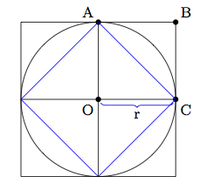# Approximating the area of a circle

Alignments to Content Standards: 7.G.A.1 7.G.B.4

Below is a picture of a circle of radius $r$ and a square of side length $r$:1. Show that $$2 \leq \frac{\text{Area(Circle)}}{\text{Area(Square)}} \leq 4.$$
2. How can we find a more accurate estimate of $\frac{\text{Area(Circle)}}{\text{Area(Square)}}$ than the one in part (a)?
3. Explain why the quotient $\frac{\text{Area(Circle)}}{\text{Area(Square)}}$ does not depend on the radius $r$.

## IM Commentary

The goal of this task is twofold:

• Use the idea of scaling to show that the ratio Area of Circle: (Radius of Circle)$^2$ does not depend on the radius.
• Use formulas for the area of squares and triangles to estimate the value of the real number $c$ satisfying $$\text{Area(Circle of Radius r)} = cr^2.$$

Additional work (refer to task here when it is ready) will show that $c = \pi$ where $\pi$ is the circumference of a circle with diameter 1.

The teacher may need to provide some guidance for part (a). Supplying the pictures in the solution should be sufficient but it would be good to let students brainstorm possible methods first before supplying the pictures. The idea of this task is taken up further in https://www.illustrativemathematics.org/illustrations/903.

## Solution

1. Below is a picture of the circle with some added line segments:The large square which surrounds the circle has area $4r^2$ because it is made up of four squares with side lengths $r$. The blue inner square has area $2r^2$ because it is made up of four triangles, each of which is half of an $r$ by $r$ square. The circle contains the blue square and is contained in the larger square so the area of the circle is larger than $2r^2$ but smaller than $4r^2$.

2. One way to get a better estimate for the area of the circle is to average the two areas found in part (b) since $2r^2$ is too small and $4r^2$ is too large. This would give $3r^2$ as an estimate. Another good method would be to put the circle on a smaller-sized grid and approximate the number of (small) squares that it contains.

3. The area of the square with side length $r$ is $r^2$. The circle with radius $r$ can be thought of as a circle of radius 1 scaled by a factor of $r$. Since the circle is a two dimensional shape scaling by $r$ will change its area by a factor of $r^2$, one factor of $r$ for each dimension. This means that \begin{align}\frac{\text{Area(Circle of radius $r$)}}{\text{Area(Square of radius $r$)}} &= \frac{r^2 \times \text{Area(Circle of radius $1$)}}{r^2} \\ &= \text{Area(Circle of radius $1$)}.\end{align}